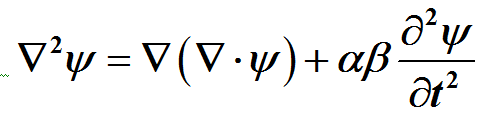# 时空阶梯理论的历史以及封顶问题History and Capping Issue of Space and Time Ladder Theory

DOI: 10.12677/MP.2016.64013, PDF, HTML, XML, 下载: 1,413  浏览: 3,355

Abstract: pace and time ladder theory makes it easier to see how space and time ladder to be found and to be completed step by step. Space and time ladder theory reveals that the origin of the universe is the Qi space-time (mc3), and we can intuitively believe that Qi space-time is straight in any direction without any bending, or zero curvature. Qi space-time results in gravitational space and time and energy space and time under Higgs mechanism, and this is the initial evolution of the universe. The polarized Qi space-time (space-time with a slight bend) = Gravitational space and time + Energy space and time, which is a relatively stable equation, and Einstein's gravitational field equation is the expression of this equation. If the evolution of the universe likes expanding the life in the same way, then this equation is like the universe stem cell. Max-well's equations have all the elements of space and time ladder theory, which is the originator of the wave equation, and its general form:. Klein-Gordon equation is the wave equation of Qi space-time, and there is no polarization of particle production. It can be said to be fluctuated between gravitational space-time, energy space and time and Qi space-time. Further polarization of Qi space-time produces the weak, electromagnetic and strong forces, and Schrodinger equation and Dirac equation describe these changes. In addition, from the beginning of the establishment of Space and time ladder theory, there is a capping problem, because it is the speed of light geometric increasing from Qi space-time (mc3), to Spirit space-time (mc9), to the imaginary space-time (mc27) and Tao space-time (mc81), and it does not seem to end. However, it was set down based on the speed of the quasar, and Tao space-time was thought to be the highest space-time. At this time, it is explored in the field of quantum mechanics, and Space and time ladder theory fits well with the Standard Model to the number of elementary particles, and furthermore, Space and time ladder theory can make a prediction: Tao space-time has 54 kinds of elementary particles, but the Standard Model gives only 36 kinds of elementary particles, so there are 18 kinds of elementary particles to be found in the Tao space-time in the future.

  常炳功. 时空阶梯的建立以及对双缝实验的解释[J]. 科技展望, 2016(1).  张玉民, 戚伯云. 电磁学(第2版)[M]. 2013(12).  爱因斯坦. 广义相对论基础[M]. 德国: 《物理年鉴》(Annalen der Physik). 1916: 769-822.  Greiner, W. (1995) Relativistic Quantum Mechanics Wave Equations.  Christianto, V. (2004) A Review of Schrödinger Equation & Classical Wave Equation.  Puppel, P. (2006) Dirac Equation and Hydrogen Atom. October 19.  张三慧. 大学基础物理学(上)(第2版)[M]. 2007(3).